# Solution assignment 07 Logarithmic functions and graphs

### Assignment 7

Draw the graph of the function: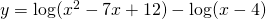### Solution

The function exists if both logarithms exist. The first is defined for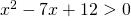, thus for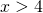or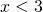. We know this because the quadratic function can be factorized: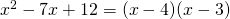The second logarithm is defined for, thus the function as a whole is defined for.

Now the function can be written as: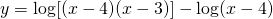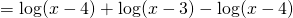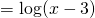We can sketch this graph more easily than the graph of the original function, but the graph is only valid for: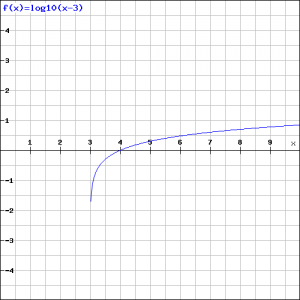0